Garrett Mayock's Blog

# Linear Regression for Predicting Time Series with Trends

### Following along Managing Supply Chain Operations Chapter 2 using Python, cont'd.Garrett Mayock posted 2019-02-28 17:46:45 UTC

As before: I am reading Managing Supply Chain Operations. Chapter 2 details Forecasting and Demand Management methods. I figured I'd kill two birds with one stone (learning the material and learning Python) by recreating the chapter material in Python. I anticipate answering the chapter exercises in Python as well, but will save that for another blog post. (Writer's note - predicting stationary time series data is covered in the previous blogs (naive, non-naive). Click here to download the Excel file I ingested.

First of all: An aside.

Let me just mention that we are getting to the territory where my appreciation for Python (and code in general) skyrockets. I took AP Stats and AP Calculus in tenth grade. That was some decade and a half ago. Unfortunately, I didn't know anything about coding then, and had to calculate everything longhand. That wasn't a lot of fun. I think everyone my age remembers the teachers justifying this by saying how we "won't always have a calculator in our pocket!" Now I have that, and a supercomputer at my fingertips - (okay, maybe not quite - my computer tests around 70-80 GFlops and at one point would have been the strongest in the world, but that point was 1993).

Hindsight is 20/20, but that's not the point - point is, I feel like I would have dived a lot further into mathematical theory if I had known about coding and permitted to use code on the exams. Who knows - I mean, I was a teenager then; teens aren't known for their robust decision-making processes. Regardless, the good part is - I'm here now, and I'm really enjoying it. I mention this right now because this textbook has pages (and an entire appendix) of explanation and formulas on how to calculate a simple ordinary-least-squares regression model, which I'm going to be able to replicate and compute with a few simple lines of code. I'm already excited.

Since the past few blogs have had a lot of explanation, I'm just going to link to explanations of topics like ordinary least squares and what factors affect product sales rather than including all the descriptions and math here.

Trending Time Series Forecasting

Let's ingest the 2.4_table_2.9 sheet:

```import pandas as pd
import matplotlib.pyplot as plt
import numpy as np

print(df)
```

The dataframe looks like this: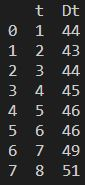The book goes on to calculate this equation: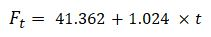Calculating simple linear regression models in Python

I calculated that eight different ways using Python, and told the results to print:

```import statsmodels.api as sm
results = sm.OLS(df['Dt'], X).fit()
print("\n StatsModels OLS summary: \n", results.summary(), "\n StatsModels OLS parameters: \n", results.params)

from sklearn.linear_model import LinearRegression
linmodel = LinearRegression().fit(X,df['Dt'])
print("\n Scikit-learn intercept and coefficient: \n",linmodel.intercept_, linmodel.coef_)

from numpy import polyfit
polmodel = polyfit(df['t'],df['Dt'],1)
print("\n NumPy 1st-degree polyfit \n", polmodel)

from scipy.stats import linregress
scimodel = linregress(df['t'],df['Dt'])
print("\n SciPy linear regression: \n", scimodel.slope, scimodel.intercept)

from scipy.optimize import curve_fit
def func(m,x,c):
y = m*x+c
return y
popt, pcov = curve_fit(func,df['t'],df['Dt'])
print("\n SciPy curve_fit: \n", popt)

from numpy.linalg import lstsq
lstsqmod = lstsq(X,df['Dt'], rcond=None)
print("\n NumPy least-squares: \n", lstsqmod)

y = np.array([df['Dt']]).T
beta_hat = np.dot(np.linalg.inv(np.dot(X.T,X)),(np.dot(X.T,y)))
print("\n Beta-hat intercept: \n", beta_hat,"\n Beta-hat slope: \n", beta_hat)

pseudo_beta_hat = np.dot(np.linalg.pinv(np.dot(X.T,X)),(np.dot(X.T,y)))
print("\n Pseudo-beta-hat intercept: \n", pseudo_beta_hat,"\n Pseudo-beta-hat slope: \n", pseudo_beta_hat)
```

Here we start with StatsModels OLS, scikit-learn's linear regression, NumPy's polyfit, SciPy's linear regression, SciPy's curve_fit, and NumPy's lstsq. See the linked pages for descriptions. The last two are simple matrix multiplication, with the latter of the two using NumPy's pseudo-inverse function, which helps find the least-squares solution to linear equation systems that lack a unique solution. All of them arrive at the same answer, of course, though some return fewer decimals.

One thing I noticed but don't have an answer for is that some of these functions assume a constant and some require a constant to be added. For example, in the functions which are fit on "X", X is actually in that case an array with two columns - a constant, and the actual df['t'] values.

Anyway, scikit-learn provides a lot of useful information in its summary function and so I'll show it here just to give you an idea of what the results look like: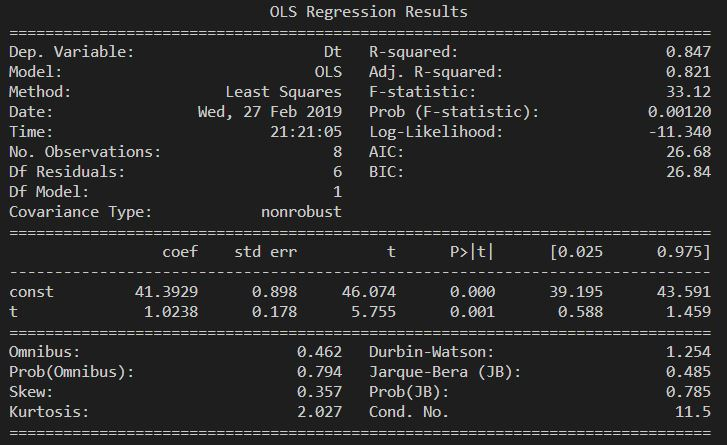We can use these results to cast a forecast out as far as we want. Let's add a forecasted values column for ten more periods:

```intercept = beta_hat
slope = beta_hat

def linRegPred(period):
y = intercept+slope*period
return y

new_pred = []
for i in range (1, len(df['t'])+11):
pred = np.round(linRegPred(i),2)
new_pred.append([i,pred])
new_pred = pd.DataFrame(new_pred, columns=['t','Ft'])

df2 = pd.merge(df,new_pred,how='outer',on=['t'])
```

That gives us forecasted values in a new column 'Ft' from periods 1 through 19. I'll plot the forecast using similar code as in the past couple blogs:

```plt.ylabel('Widget Demand (000s)');
plt.ylim(0,75);
plt.axes().yaxis.grid(linestyle=':');
plt.xlabel('Time Period');
plt.xlim(0,18);
plt.title('Widget Demand Data');
plt.plot(df2['t'], df2['Ft'], marker='D');
plt.plot(df2['t'], df2['Dt'], marker='o');
plt.legend();
plt.show();
```

Which results in: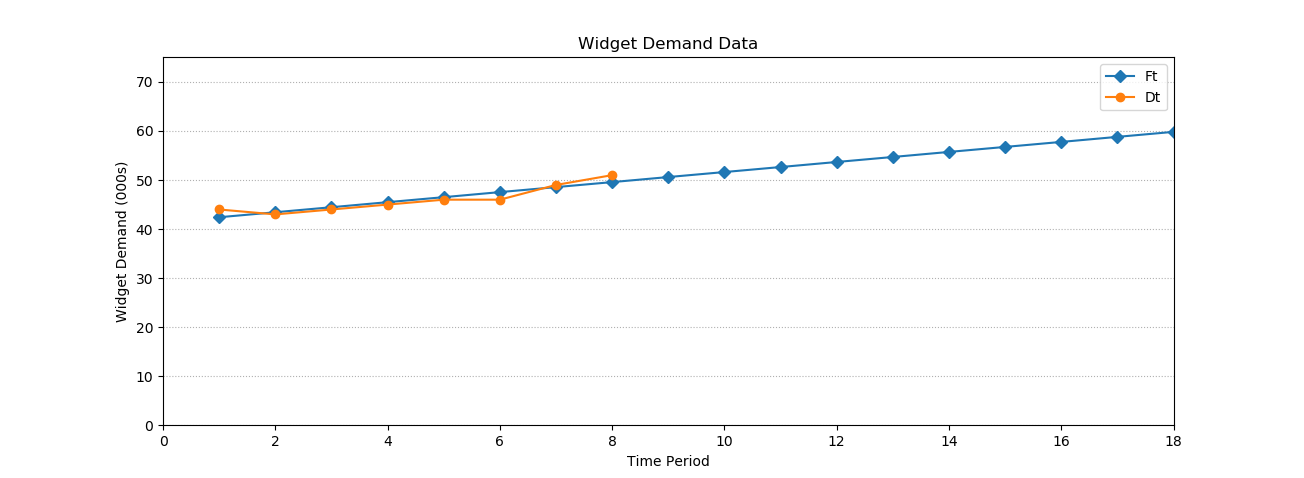Calculating regression coefficients in Excel

The book also shows how to calculate regression coefficients using Excel's Analysis ToolPak add-in. See: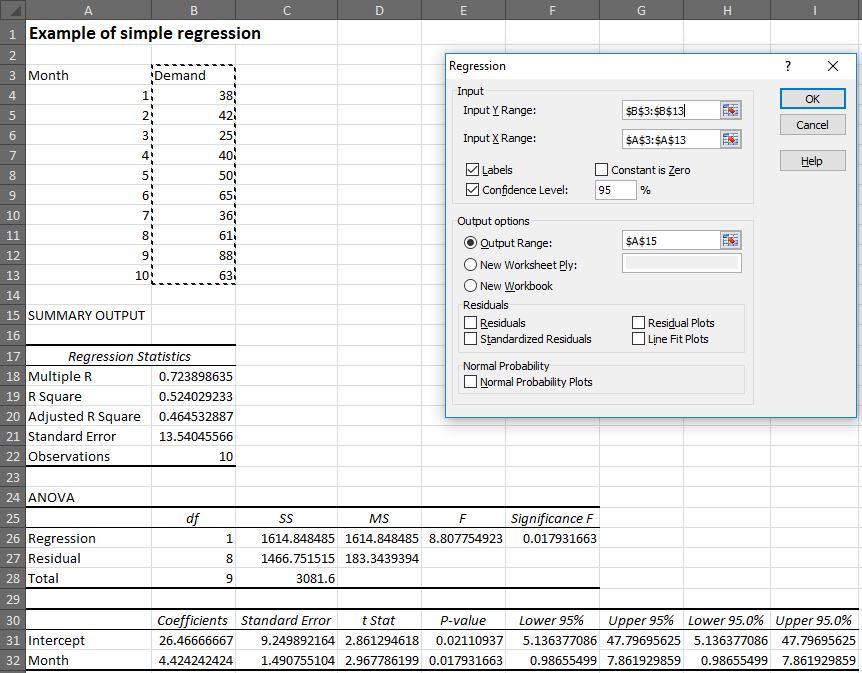Plotting the results in Python

I'll pull this in Python and plot it, too:

```df = pd.read_excel('filepath/chapter_2_instruction.xlsx', sheet_name='2.4_figure_2.13', header=2, index_col=None, nrows=10, usecols=[0,1])

# This calculates the function intercepts
import statsmodels.api as sm
y = np.array([df['Demand']]).T
beta_hat = np.dot(np.linalg.inv(np.dot(X.T,X)),(np.dot(X.T,y)))
intercept = beta_hat
slope = beta_hat

# This defines the function
def linRegPred(period):
y = intercept+slope*period
return y

# This calls the function to create forecasted values
new_pred = []
for i in range (1, len(df['Month'])+11):
pred = np.round(linRegPred(i),2)
new_pred.append([i,pred])
new_pred = pd.DataFrame(new_pred, columns=['Month','Forecast'])

# This merges the new dataframe (the one with forecasts) with the old
df2 = pd.merge(df,new_pred,how='outer',on=['Month'])
print(df2)

# This plots the demand and the linear regression algorithm's predicted demand
plt.ylabel('Widget Demand (000s)');
plt.ylim(0,120);
plt.axes().yaxis.grid(linestyle=':');
plt.xlabel('Month');
plt.xlim(0,18);
plt.title('Widget Demand Data');
plt.plot(df2['Month'], df2['Forecast'], marker='D');
plt.plot(df2['Month'], df2['Demand'], marker='o');
plt.legend();
plt.show();
```

Which results in: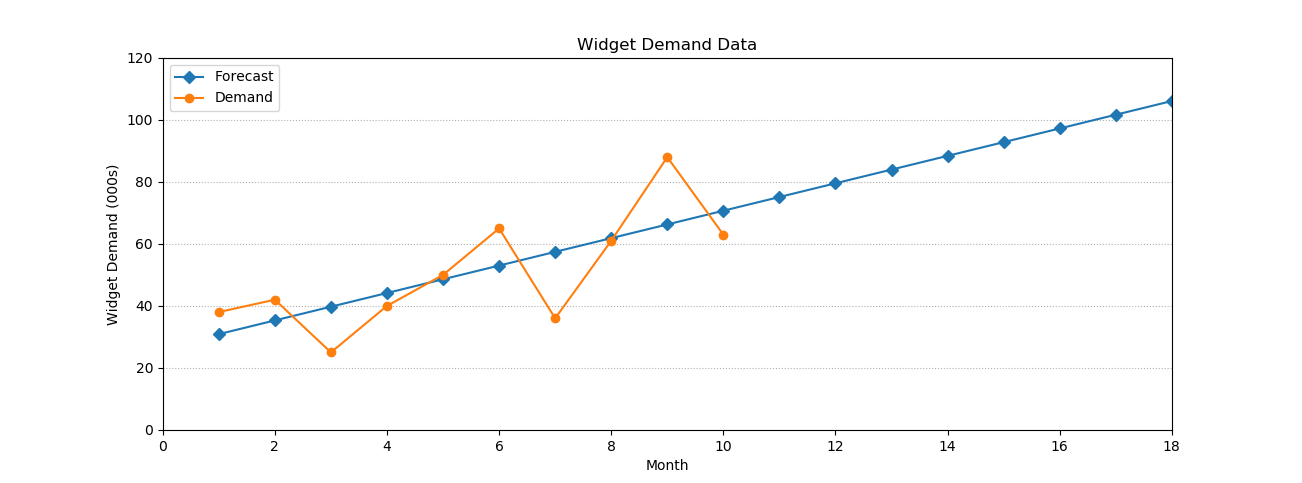Next Steps

That's it for regression analysis! Holt's Trend Model will be used in the next post.